# Mathematical Symbols: List & Meanings

Lesson Transcript
Instructor: Glenda Boozer
Mathematical symbols are used to understand mathematical expressions easily. Explore the list and meanings of mathematical symbols involving relation, basic arithmetic, grouping, powers, roots, special numbers, miscellaneous, and calculus. Updated: 10/12/2021

## Math Is a Language of Symbols

Imagine that you have a pet hamster that hasn't stopped growing since the day you got it. To track and analyze its amazing growth, you'll need to use a range of mathematical symbols. In math, symbols are concise ways to show mathematical relations and operations.

In this lesson, we'll stick with the symbols that you'll see most often. Remember, if all else fails, you can always write things out in words, but make sure to ask someone about the correct symbol later.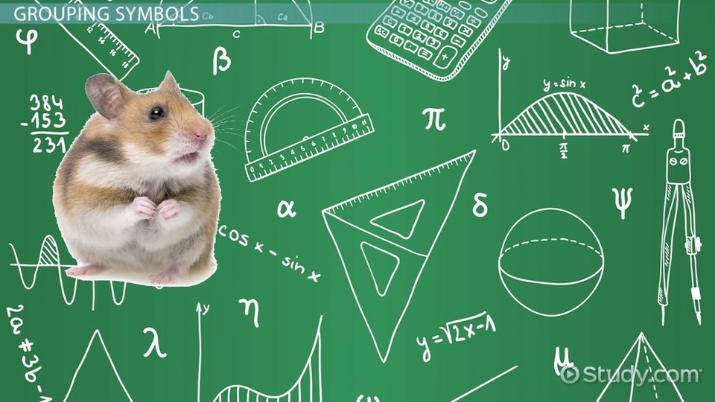An error occurred trying to load this video.

Try refreshing the page, or contact customer support.

Coming up next: Arithmetic with Whole Numbers

### You're on a roll. Keep up the good work!

Replay
Your next lesson will play in 10 seconds
• 0:34 Relation Symbols and…
• 3:03 Grouping Symbols
• 3:59 Powers and Roots
• 4:43 Special Numbers and…
• 5:58 Symbols Used in Calculus
• 7:13 Lesson Summary
Save Save

Want to watch this again later?

Timeline
Autoplay
Autoplay
Speed Speed

## Relation Symbols

To get a true idea of your giant hamster's scale, you'll need to show how its size relates to other quantities. Relation symbols tell us how one expression, a mathematical representation of a quantity, relates to another expression. You probably know the equal sign: =. It tells us that the expressions on both sides of it are exactly equal to one another. So, you could say your hamster's weight equals 205 pounds.

While that gives an exact value for its weight, you could use this sign, which means that the expressions are only approximately equal: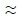For example, your hamster may be approximately equal in size to a baby elephant.

When things are not equal, one is greater than the other, so we use greater than and less than signs.

• >
• <

These show which expression is less: each symbol points to the lesser expression.

These symbols, which are similar to the greater than and less than signs, are the greater than or equal to and less than or equal to signs: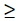These signs include the amount itself, whereas greater than or less than refer to numbers right up to, but not including, that exact amount. Think of the difference between your hamster being at least 200 pounds and greater than 200 pounds.

## Basic Arithmetic

As your hamster continues its alarming growth, you'll need to know basic arithmetic symbols. We know that + means addition, and - means subtraction, and you can use these symbols to show how its size has changed.

But, what if you wanted to convert its weight from pounds to another unit of measurement? In that case, you'd use multiplication or division. However, we can't always use this common multiplication symbol because it may sometimes be confused with the variable x: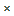Instead we use the asterisk: *

Or we can use a dot:

Similarly, we can either use this symbol to represent division: ÷ or a slash /.

Along with these goes this symbol that stands for either adding or subtracting the value that comes after it: ±. If you noticed that your hamsters eats about 40 pounds of cabbage a day, but sometimes he eats 35 or 45 pounds, then you could say that he eats 40 ± 5 pounds a day.

## Grouping Symbols

As your hamster calculations become increasingly complex, you'll need grouping symbols to help you figure out the order of operations.

• Parentheses look like this: ( ).
• Square brackets look like this: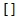• Curly brackets look like this: { }

All of them are used to group items together. Grouping symbols are used to tell you to do the math of the items in the group first. The curly and square brackets are generally used to group items that are already in parentheses. Grouping symbols may also be used to indicate multiplication.

Parentheses also have some specialized meanings. When we write f(x), this does not mean f times x. It means the value of the function f when the input value is x. Parentheses are also used to show the coordinates of a point on a graph, like this: (3,2). When we write 3,2 we are making a short list with the numbers 3 and 2.

## Powers and Roots

Hopefully, your hamster isn't experiencing exponential growth. Just in case, we can raise an expression to any power by using the exponent symbol, which looks like this: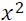The small number is the exponent. This example means x-squared.

Roots are the opposite of powers: 3 is the square root of 9 because 3^2 = 9, -2 is the cube root of -8 because (-2)^3 = -8 and 2 is the fifth root of 32 because 2^5 = 32. The square root image looks like this: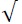And the cube root looks like this: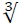We can also have fourth roots, fifth roots and so on.

To unlock this lesson you must be a Study.com Member.

### Register to view this lesson

Are you a student or a teacher?